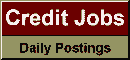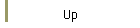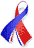DefaultRisk.com the web's biggest credit risk modeling resource.doi> search: A or B Export citation to:- HTML- Text (plain)- BibTeX- RIS- ReDIF

Recursive Evaluation of a Family of Compound Distributions

by Harry H. Panjer of the University of Waterloo

July 1981

Introduction: Compound distributions such as the compound Poisson and the compound negative binomial are used extensively in the theory of risk to model the distribution of the total claims incurred in a fixed period of time. The usual method of evaluating the distribution function requires the computation of many convolutions of the conditional distribution of the amount of a claim given that a claim has occurred. When the expected number of claims is large, the computation can become unwieldy even with modern large scale electronic computers.

In this paper, a recursive definition of the distribution of total claims is developed for a family of claim number distributions and arbitrary claim amount distributions. When the claim amount is discrete, the recursive definition can be used to compute the distribution of total claims without the use of convolutions. This can reduce the number of required computations by several orders of magnitude for sufficiently large portfolios.

Results for some specific distributions have been previously obtained using generating functions and Laplace transforms (see PANJER (1980)[REF] including discussion). The simple algebraic proof of this paper yields all the previous results as special cases.

Published in: Astin Bulletin, Vol. 12, No. 1, (July 1981), pp. 22-26.